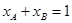# Define molarity, molality, normality, mole-fraction.### Molarity (M):

Molarity is defined as the number of moles of solute per litter of solution. That is,Here,
W = weight of solute.
M = molecular mass of solute
V = volume of solution

### Molality (m):

It is defined as the number of moles of solute per kg of solvent. That is,Here,
b = weight of solvent

### Normality (N):

It is defined as the number of gram equivalents of the solute dissolved per litter of the solution at a specific temperature.### Mole-fraction (x):

Mole fraction is the ratio of the number of moles of solute and the total number of moles of solute and solvent. The mole fraction of any constituent of a solution is defined as the number of moles of that constituent per mole of the solution. If the solution contained molesof A andmoles of B, thenand